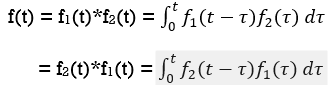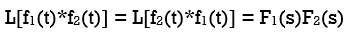Goseeko blog# What is the Convolution Theorem?

The convolution theorem states that f1(t) and f2(t) are two time functions which are zero for t<0 and if Laplace transform are F1(s) and F2(s)then the transform of convolution of f1(t) and f2(t) is the product of individual transforms F1(s) and F2(s).The Laplace transform isThe Laplace Transformation transforms convolution into multiplication.

The graphical presentation of the convolution integral helps in the understanding of every step in the convolution procedure. According to the definition integral, the convolution procedure involves the following steps:

• The interval in which convolution is equal to zero is identified by applying the convolution duration property.
• Firstly, fold one of the signals which is simpler amongst two along the vertical axis. Then this signal represents one signal in the time scale.
• Now shift the signal from left to right and vary parameters from -∞ to +∞. Then check for the overlapping area in the two signals.
• Then find the integral product of those signals in the respective given intervals.

In a network analysis we apply convolution theorem. With the help of Laplace transform we proceed as convolution in the time domain is multiplication in S domain. This makes the calculation a bit easier.

Interested in learning about similar topics? Here are a few hand-picked blogs for you!